# The SURVEYFREQ Procedure

#### Wald Log-Linear Chi-Square Test

If you specify the WLLCHISQ option in the TABLES statement, PROC SURVEYFREQ computes a Wald test for independence based on the log odds ratios. See the section Wald Chi-Square Test for more information about Wald tests.

For a two-way table of R rows and C columns, the Wald log-linear test is based on the (R – 1)(C – 1)-dimensional array of elements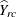,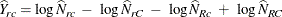where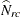is the estimated total for table cell (r, c). The null hypothesis of independence between the row and column variables can be expressed as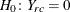for all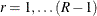and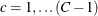. This null hypothesis can be stated equivalently in terms of cell proportions.

The generalized Wald log-linear chi-square statistic is computed as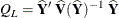where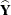is the (R – 1)(C – 1)-dimensional array of the, and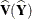estimates the variance of,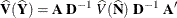where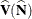is the covariance matrix of the estimates, which is computed as described in the section Covariance of Totals.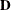is a diagonal matrix with the estimated totalson the diagonal, and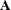is the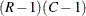by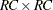linear contrast matrix.

Under the null hypothesis of independence, the statistic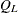approximately follows a chi-square distribution with (R – 1)(C – 1) degrees of freedom for large samples.

PROC SURVEYFREQ computes the Wald log-linear F statistic as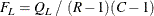Under the null hypothesis of independence,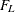approximately follows an F distribution with (R – 1)(C – 1) numerator degrees of freedom. PROC SURVEYFREQ computes the denominator degrees of freedom as described in the section Degrees of Freedom. Alternatively, you can specify the denominator degrees of freedom with the DF= option in the TABLES statement.

For tables larger than, PROC SURVEYFREQ also computes the adjusted Wald log-linear F statistic as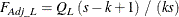where k = (R – 1)(C – 1), and s is the denominator degrees of freedom, which is computed as described in the section Degrees of Freedom. Alternatively, you can specify the value of s with the DF= option in the TABLES statement. Note that fortables, k = (R – 1)(C – 1) = 1, and therefore the adjusted Wald F statistic equals the (unadjusted) Wald F statistic, with the same numerator and denominator degrees of freedom.

Under the null hypothesis,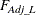approximately follows an F distribution with k numerator degrees of freedom and (sk + 1) denominator degrees of freedom.